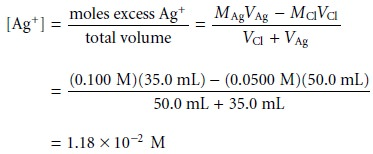Home | | Modern Analytical Chemistry | Precipitation Titration Curves

# Precipitation Titration Curves

The titration curve for a precipitation titration follows the change in either the ana- lyteâ€™s or titrantâ€™s concentration as a function of the volume of titrant.

Titration Curves

The titration curve for a precipitation titration follows the change in either the ana- lyteâ€™s or titrantâ€™s concentration as a function of the volume of titrant. For example, in an analysis for Iâ€“ using Ag+ as a titrant

Ag+(aq)+ Iâ€“(aq) < = = = = > AgI(s)

the titration curve may be a plot of pAg or pI as a function of the titrantâ€™s volume. As we have done with previous titrations, we first show how to calculate the titra- tion curve and then demonstrate how to quickly sketch the titration curve.

## Calculating the Titration Curve

As an example, letâ€™s calculate the titration curve for the titration of 50.0 mL of 0.0500 M Clâ€“ with 0.100 M Ag+. The reaction in this case is

Ag+(aq)+ Clâ€“(aq) < = = = = > AgCl(s)

The equilibrium constant for the reaction is

K = (Ksp)â€“1 = (1.8 x 10â€“10)â€“1 = 5.6 x 109

Since the equilibrium constant is large, we may assume that Ag+ and Clâ€“ react completely.

By now you are familiar with our approach to calculating titration curves. The first task is to calculate the volume of Ag+ needed to reach the equivalence point. The stoichiometry of the reaction requires thatshows that we need 25.0 mL of Ag+ to reach the equivalence point.

Before the equivalence point Clâ€“ is in excess. The concentration of unreacted Clâ€“ after adding 10.0 mL of Ag+, for example, isIf the titration curve follows the change in concentration for Clâ€“, then we calculate pCl as

pCl = â€“log[Clâ€“] = â€“log(2.50 x 10â€“2) = 1.60

However, if we wish to follow the change in concentration for Ag+ then we must first calculate its concentration. To do so we use the Ksp expression for AgCl

Ksp = [Ag+][Clâ€“] = 1.8 x 10â€“10gives a pAg of 8.14.

At the equivalence point, we know that the concentrations of Ag+ and Clâ€“ are equal. Using the solubility product expressionAt the equivalence point, therefore, pAg and pCl are both 4.89.

After the equivalence point, the titration mixture contains excess Ag+. The con- centration of Ag+ after adding 35.0 mL of titrant isor a pCl of 7.82. Additional results for the titration curve are shown in Table 9.21 and Figure 9.41.## Sketching the Titration Curve

As we have done for acidâ€“base, complexometric titrations, and redox titrations, we now show how to quickly sketch a precipitation titration curve using a minimum number of calculationsStudy Material, Lecturing Notes, Assignment, Reference, Wiki description explanation, brief detail
Modern Analytical Chemistry: Titrimetric Methods of Analysis : Precipitation Titration Curves |